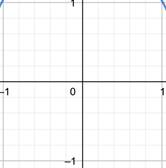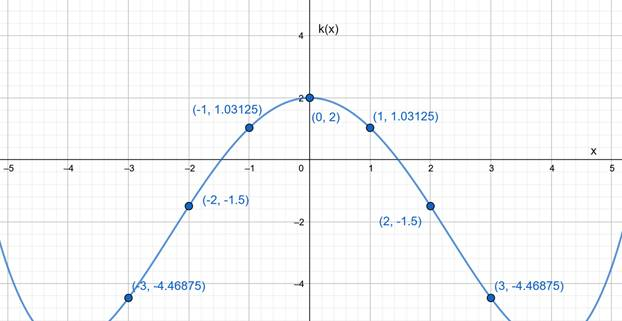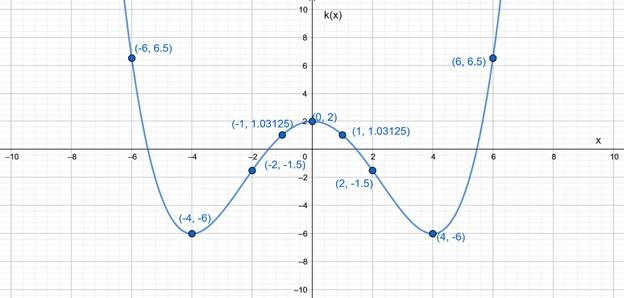# To graph : The function in a specified viewing rectangle.### Precalculus: Mathematics for Calcu...

6th Edition
Stewart + 5 others
Publisher: Cengage Learning
ISBN: 9780840068071### Precalculus: Mathematics for Calcu...

6th Edition
Stewart + 5 others
Publisher: Cengage Learning
ISBN: 9780840068071

#### Solutions

Chapter 2.2, Problem 32E

a.

To determine

## To graph : The function in a specified viewing rectangle.

Expert Solution

### Explanation of Solution

Given information :

k(x)=132x4x2+2

The viewing rectangle is [1,1] by [1,1]

Graph :

 x 0 1 −1 k(x) 2 1.03125 1.03125This is the graph (in fact blank) of given function represented in the viewing rectangle [1,1] by [1,1] .

Interpretation : In the viewing rectangle [1,1] by [1,1] , K(x) has no value.

b.

To determine

Expert Solution

### Explanation of Solution

Given information :

k(x)=132x4x2+2

The viewing rectangle is [2,2] by [2,2] .

Graph :

 x 0 1 2 −1 −2 k(x) 2 1.03125 −1.5 1.03125 −1.5This is the graph of given function represented in the viewing rectangle of [2,2] by [2,2] .

Interpretation : The graph of the given function is represented in the viewing rectangle [2,2] by [2,2]. This graph shows the more appropriate view of the function than the graph shown in the viewing rectangle [1,1] by [1,1] .

c.

To determine

Expert Solution

### Explanation of Solution

Given information :

k(x)=132x4x2+2

The viewing rectangle is [5,5] by [5,5] .

Graph :

 x 0 1 2 3 −1 −2 −3 k(x) 2 1.03125 −1.5 −4.46875 1.03125 −1.5 −4.46875This is the graph of given function represented in the viewing rectangle of [5,5] by [5,5] .

Interpretation : The graph of the given function is represented in the viewing rectangle [5,5] by [5,5] . This graph shows more appropriate view of the function than the graph shown in the viewing rectangle [2,2] by [2,2] .

d.

To determine

Expert Solution

### Explanation of Solution

Given information :

k(x)=132x4x2+2

The viewing rectangle is [10,10] by [10,10] .

Graph :

 x 0 1 2 4 6 −1 −2 −4 −6 k(x) 2 1.03125 −1.5 −6 6.5 1.03125 −1.5 −6 6.5This is the graph of given function represented in the viewing rectangle of [10,10] by [10,10]

Interpretation : The graph of the given function is represented in the viewing rectangle [10,10] by [10,10] . This graph represents more appropriate view than the graph shown in the viewing rectangle [5,5] by [5,5] . Therefore, this graph represents the most appropriate view among the four.

### Have a homework question?

Subscribe to bartleby learn! Ask subject matter experts 30 homework questions each month. Plus, you’ll have access to millions of step-by-step textbook answers!